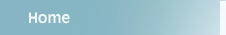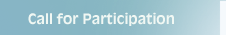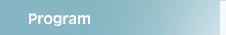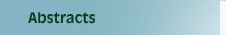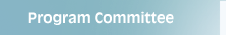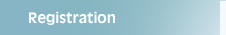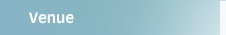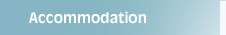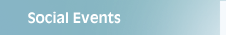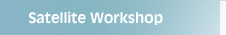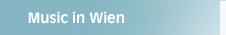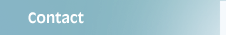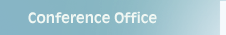Olivier Carton, Liafa - Universite Paris Diderot Minimal Automata   The talk highlights some points of the chapter, in particular the relation between the Moore and the Hopcroft minimization algorithms. Recent results on extensions of Hopcroft's algorithm, and on average complexity are also reported. We discuss also practical applications to linguistics. Manfred Droste, Universität Leipzig Weighted Automata We investigate weighted automata and their relationship to weighted logics. For this, we present syntax and semantics of a quantitative logic; the semantics counts 'how often' a formula is true in a given word. Our main result, extending the classical result of Büchi, shows that if the weights are taken from an arbitrary semiring, then weighted automata and a syntactically defined fragment of our weighted logic are expressively equivalent. A corresponding result holds for infinite words. Moreover, this extends to weighted automata with (non-semiring) average-type behaviors, or with discounting or limit average objectives for infinite words. Søren Eilers, University of Copenhagen Classification of Symbolic Dynamical Systems   Symbolic dynamics is part of dynamical systems theory. It studies discrete dynamical systems called *shift spaces* and their relations under appropriately defined morphisms, in particular isomorphisms called conjugacies. There is a considerable overlap between symbolic dynamics and automata theory. Actually, one of the basic objects of symbolic dynamics,  the sofic system, is essentially the same as a finite automaton. In addition, the morphisms of shift spaces are a particular case of rational transductions, that is functions defined by finite automata with output. The difference is that symbolic dynamics considers mostly infinite words and that all states of the automata are initial and final. The origins of symbolic dynamics has motivated and allowed for a deep and rich classification theory of shift spaces by algebraic invariants. The most fundamental classification theory -- initiated by Williams -- famously addresses conjugacy of so-called shifts of finite type, but has unearthed deep complications which seem to prohibit a deterministic classification in the sofic case. Thus, a new emphasis has evolved on classification up to coarser relations, of which we focus in particular on the notion of *flow equivalence* and present new results obtained with Boyle and Carlsen. Zoltán Ésik, University of Szeged  Equational Theories for Automata The validity of several constructions on automata only depends on a few equational properties of their behavior. In the talk, we will give complete desriptions of these equational properties and show some of their applications. Javier Esparza, Universität Stuttgart Software Model Checking with Automata We present the algorithms behind jMoped, a model-checker for Java programs. jMoped uses automata theory to model Java programs (as symbolic pushdown systems), to obtain basic algorithms for the verification problem (using results on prefix rewriting going back to Büchi), and to design good data structures for the problem (automata with automata as transition labels). Tero Harju, University of Turku Finite Transducers and Rational Transductions Finite transducers are generalized finite automata with output. Their history in the modern setting goes back to the end of 1950’s when Rabin and Scott published their study on the topic. One of the basic results of the chapter characterizes rational transductions realized by finite transducers in terms of compositions of morphisms. This result arises from Nivat’s theorem, which was extended in the early 1980s to purely morphic representations by Culik, Fich and Salomaa and later improved by several authors. We also consider an impressive application of transductions due to Kari on aperiodic tilings of the plane. Also, transductions are used to solve the isomorphism problem of F-semigroups. Finally, we study decision problems for transducers and their transductions by establishing several rather simply formulated natural undecidable problems. In this context especially problems related to finite substitutions are considered. Undecidability of the equivalence problem for finite transductions, due to Lisovik and Ibarra, is stated and sharpened in many steps to a “final” form, due to Kunc. Thomas A. Henzinger, IST Austria, Klosterneuburg Weighted Automata over Infinite Words We survey known complexity and expressiveness results about weighted automata with limsup and limit average objectives.  These automata prove useful in the verification of resource properties for reactive systems. Michal Kunc, Masaryk University, Brno Language Equations The lecture will provide a survey of the known results on equations where unknowns are sets of words and one of the basic operations is elementwise concatenation. The emphasis will be put on classifying systems of language equations according to the methods of research and on comparing similar properties of different families. While explicit systems are fundamental for the theory of formal grammars since they represent semantics of context-free grammars and their natural variants, implicit systems are notable for the computational completeness of systems of extremely simple forms. Christof Löding, RWTH Aachen Automata on Infinite Trees Automata on infinite trees have been introduced by Rabin at the end of the sixties to generalize Büchi's result on the decidability of the monadic second-order theory of the natural numbers with successor function to the structure with two successor functions - the infinite binary tree. Since then a rich theory of these automata has been developed, including further applications in logic and synthesis, and the connection to games of infinite duration. The aim of this talk is to give an overview of the classical main results on automata on infinite trees, as well as to present some more recent work on special types of automata and partial results on the parity index problem. Hermann Maurer, Technische Universität Graz Long Range Forecasting is Necessary but Impossible In this talk I will explain why we need long-range forecasts, but also why correct predictions are almost impossible: indeed some of the reasons are more subtle than is generally understood. As an outcome of my arguments it will be clear that we are in not just for some further changes, but for dramatic ones. One reason, but not the only one, is the development of networked information. It will be important to determine how much information and knowledge we will be able to "externalize", i.e. to move from our brains into computer networks and data bases without destroying our capacity of coherent thinking. I will add a few samples of  the radical changes we will be confronted with, including some novel pictures and video-clips. Nicole Schweikardt, Goethe-Universität Frankfurt am Main Automata in Document Processing The applications of automata in document processing include, for example, basic algorithms for searching for a keyword or a match for a regular expression, validation of XML documents, and query evaluation on XML documents. Automata theoretic techniques also provide powerful tools for determining the expressive power and the computational complexity of various languages for querying XML documents. In this talk I want to give an overview of the various applications of automata in the area of document processing. Olivier Serre, LIAFA, CNRS, Paris Recursions Schemes and their Automata Models In this talk, I will present two equi-expressive models for generating infinite trees. The first one are higher-order recursion schemes, which can be though as a deterministic rewriting system over terms (essentially, the simply-typed lambda calculus with recursion). The second one are higher-order pushdown automata, which are an extension of pushdown automata that uses stacks of stacks instead of stacks of symbols. The first part of the talk will be devoted to present the models and give examples. The second part, will focus on properties of the trees generated by such models (in particular, the decidability of the monadic second order logic), and will illustrate the advantages of automata techniques when working with such objects. Pedro Silva, University Porto Automorphism Problems in the Free Group of Rank 2   Automorphisms of free groups constitute a major subject of research in combinatorial group theory. Many natural problems remain open for free groups of arbitrary finite rank, but the peculiarities of the rank 2 case have allowed positive solutions in several cases. In this talk, we shall consider two recent examples of problems where algebra, automata theory and combinatorics on words are combined to obtain results. A first example is given by the mixed orbit problem. Given elements u, v ∈ Fr, it is decidable (Whitehead, 1936) whether or not v = u φ  for some φ ∈ AutFr. Given finitely generated subgroups H,K ≤f.g. Fr, it is also decidable (Gersten, 1984) whether or not K = Hφ for some φ ∈ AutFr. However, it remains an open problem to decide, given u ∈ Fr and H ≤f.g. Fr, whether or not uφ ∈ H for some φ ∈ AutFr. In the rank 2 case, decidability can be proved using a strong theorem on decidability of systems of equations with rational constraints (Diekert, Gutierrez and Hagenah, 2005). However, an alternative automata-theoretic approach based on the dynamical study of the orbits of Stallings’ automata allows the introduction of constraints in the type of automorphisms and the obtention of more general results. Such results were obtained in joint work with Pascal Weil. A second example concerns the cost of inverting an automorphism. If Fr = , a natural norm can be defined on AutFr through ||φ||1 = |a1φ| + · · · + |arφ|. How can we bound ||φ−1||1 in terms of ||φ||1? The usual decomposition of an automorphisms in terms of the elementary Whitehead automorphisms provides only an exponential bound at first sight. We can prove that in F2 the (asymptotical) cost of inversion is precisely quadratic and that this is no longer true in F3. These results were obtained in joint work with Manuel Ladra and Enric Ventura. Jeffrey Shallit, University of Waterloo Enumeration of Languages, Automata, and Regular Expressions The exact enumeration of finite automata, the regular languages they accept, and regular expressions that specify regular languages is a challenging problem that is still not fully understood.  In this talk I will survey what is known, describe some general techniques, and discuss what remains to be done. Wolfgang Thomas, RWTH Aachen Automata on Finite Trees The basic theory of automata on finite trees can be developed in very close analogy to the classical theory of automata on words. On the other hand, several questions turn out to be very hard when generalizing from words to trees, and new phenomena have to be addressed. We give a survey on these questions, focussing on recent progress in the classification of regular tree languages and on the use of finite tree automata in infinite-state system verification. Mikhail Volkov, Ural State University, Ekaterinburg Cerny's Conjecture and the Road Coloring Problem We discuss relations between synchronizing automata and primitive digraphs. In particular, we present several infinite series of synchronizing automata for which the minimum length of reset words is close to the square of the number of states. These automata are closely related to primitive digraphs with large exponent. Igor Walukiewicz, LaBRI, CNRS - Université de Bordeaux The Synthesis Problem We will discuss the problem of synthesising a reactive system.  The most standard instance of this problem asks to construct a finite input-output automaton satisfying a given regular specification. During fifty years since its introduction by Church, numerous extensions of the initial formulation have been considered. One particularly challenging case is that of distributed synthesis where a construction of a network of input/output automata is required. We will start from the original formulation of Church, and another classical setting of Ramadge and Wonham introduced 30 years later. We will explain how the tools introduced for the former problem can be used to solve the later. Then we will present the challenge of distributed synthesis and how the theory of traces can help in finding a satisfactory formulation of the synthesis problem. Thomas Wilke, Christian-Albrechts-Universität zu Kiel Functional Programs for Regular Expression Matching We develop an elegant Haskell program for matching regular expressions: (i)   the program is purely functional; (ii) it is overloaded over arbitrary  semirings, which not only allows to solve the ordinary matching problem but  also supports other applications like computing leftmost longest matchings  or the number of matchings, all with a single algorithm; (iii) it is more powerful than one would expect, as it can be used for parsing every  context-free language by taking advantage of laziness. The developed program is based on an old technique to turn regular expressions into finite automata which makes it efficient both in terms of worst case time and space bounds and actual performance: despite its simplicity, the Haskell implementation can compete with a recently published C++ program for the same problem.